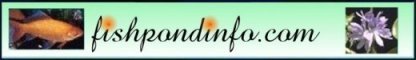FTC Disclosure: Fishpondinfo contains affiliate links, and, if you click on such a link and make a purchase, and I meet their minimal requirements, then I will be compensated.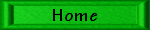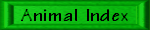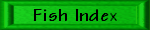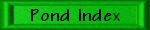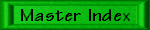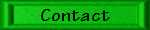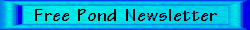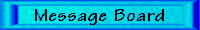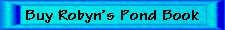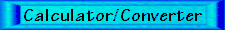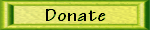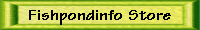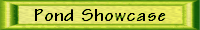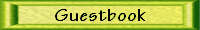# My Nano Reefs Part Twenty-Three - 7/16 to 12/17

Last Updated: 3/21/19

On 7/2/16, the salinities were 1.025, 1.025, and 1.025 for the 12 and 6 gallon tanks and the make-up water. The 12 gallon's thermometer read 84 degrees F, and the 6 gallon's thermometer read 80 degrees F.

On 7/9/16, the salinities were 1.025, 1.025, and 1.025 for the 12 and 6 gallon tanks and the make-up water. The 12 gallon's thermometer read 84 degrees F, and the 6 gallon's thermometer read 80 degrees F. Yes, it IS the same three weeks in a row!

I cleaned the tanks on 7/16/16. All three salinities were 1.025.

On 7/23/16, the salinities were 1.025, 1.0255, and 1.0255 for the 12 and 6 gallon tanks and the make-up water. The 12 gallon's thermometer read 84 degrees F, and the 6 gallon's thermometer read 80 degrees F.

On 7/31/16, the salinities were 1.0255, 1.026, and 1.025 for the 12 and 6 gallon tanks and the make-up water. The 12 gallon's thermometer read 84 degrees F, and the 6 gallon's thermometer read 80 degrees F.

On 8/6/16, the salinities were 1.025, 1.02, and 1.0255 for the 12 and 6 gallon tanks and the make-up water. The 12 gallon's thermometer read 84 degrees F, and the 6 gallon's thermometer read 80 degrees F.

On 8/13/16, I did the usual tank cleanings. All three waters were at a SG of 1.025.

On 8/20/16, the salinities were 1.025, 1.025, and 1.025 for the 12 and 6 gallon tanks and the make-up water. The 12 gallon's thermometer read 84 degrees F, and the 6 gallon's thermometer read 80 degrees F.

On 8/27/16, the salinities were 1.025, 1.025, and 1.025 for the 12 and 6 gallon tanks and the make-up water. The 12 gallon's thermometer read 84 degrees F, and the 6 gallon's thermometer read 80 degrees F. Yep, the same.

On 9/3/16, the salinities were 1.025, 1.025, and 1.025 for the 12 and 6 gallon tanks and the make-up water. The 12 gallon's thermometer read 84 degrees F, and the 6 gallon's thermometer read 80 degrees F. Yep, the same.

On 9/10/16, the salinities were 1.025, 1.025, and 1.025 for the 12 and 6 gallon tanks and the make-up water. The 12 gallon's thermometer read 84 degrees F, and the 6 gallon's thermometer read 80 degrees F. Yep, the same.

On 9/17/16, the salinities were 1.025, 1.025, and 1.025 for the 12 and 6 gallon tanks and the make-up water. The 12 gallon's thermometer read 84 degrees F, and the 6 gallon's thermometer read 80 degrees F. Yep, the same.

On 9/24/16, the salinities were 1.025, 1.025, and 1.025 for the 12 and 6 gallon tanks and the make-up water. The 12 gallon's thermometer read 84 degrees F, and the 6 gallon's thermometer read 80 degrees F. Yep, the same.

On 10/1/16, the salinities were 1.025, 1.026, and 1.025 for the 12 and 6 gallon tanks and the make-up water. The 12 gallon's thermometer read 82 degrees F, and the 6 gallon's thermometer read 78 degrees F. Oh, it changed!

On 10/8/16, the salinities were 1.025, 1.025, and 1.0255 for the 12 and 6 gallon tanks and the make-up water. The 12 gallon's thermometer read 84 degrees F, and the 6 gallon's thermometer read 80 degrees F. Why am I doing this?

On 10/15/16, the salinities were 1.025, 1.025, and 1.025 for the 12 and 6 gallon tanks and the make-up water. The 12 gallon's thermometer read 80 degrees F, and the 6 gallon's thermometer read 80 degrees F. Why am I still doing this?

On 10/22/16, the salinities were 1.025, 1.025, and 1.025 for the 12 and 6 gallon tanks and the make-up water. The 12 gallon's thermometer read 82 degrees F, and the 6 gallon's thermometer read 78 degrees F.

On 10/29/16, the salinities were 1.025, 1.025, and 1.025 for the 12 and 6 gallon tanks and the make-up water. The 12 gallon's thermometer read 80 degrees F, and the 6 gallon's thermometer was on the ground, and I didn't read it.

On 11/5/16, the salinities were 1.025, 1.025, and 1.025 for the 12 and 6 gallon tanks and the make-up water. The 12 gallon's thermometer read 80 degrees F, and the 6 gallon's thermometer read 80 degrees F.

On 11/12/16, the salinities were 1.025, 1.025, and 1.025 for the 12 and 6 gallon tanks and the make-up water.

On 11/19/16, the salinities were 1.025, 1.025, and 1.025 for the 12 and 6 gallon tanks and the make-up water.

On 11/25/16, I was near CTE Aquatics and decided to stop in. I ended up buying a neon goby for my 6 gallon nano reef (and three wood shrimp for my 50 gallon freshwater tank).

On 11/26/16, the salinities were 1.025, 1.025, and 1.025 for the 12 and 6 gallon tanks and the make-up water. I lifted up the now detached glob of green star polyps on the left top of the 12 gallon nano reef and moved them towards the wall to try to get them off of my poor brain coral. They keep stinging the brain coral which has started to bleach on one side.

On 12/3/16, the salinities were 1.025, 1.025, and 1.025 for the 12 and 6 gallon tanks and the make-up water.

On 12/10/16, the salinities were 1.025, 1.025, and 1.025 for the 12 and 6 gallon tanks and the make-up water. The 12 gallon's thermometer read 80 degrees F.

On 12/17/16, the salinities were 1.025, 1.025, and 1.025 for the 12 and 6 gallon tanks and the make-up water. The 12 gallon's thermometer read 78 degrees F, and the 6 gallon's thermometer read 76 degrees F.

On 12/24/16, the salinities were 1.025, 1.025, and 1.025 for the 12 and 6 gallon tanks and the make-up water. The 12 gallon's thermometer read 78 degrees F, and the 6 gallon's thermometer read 77 degrees F.

On 12/31/16, the salinities were 1.025, 1.025, and 1.025 for the 12 and 6 gallon tanks and the make-up water.

On 1/7/17, the salinities were 1.025, 1.025, and 1.025 for the 12 and 6 gallon tanks and the make-up water. The 12 gallon's thermometer read 78 degrees F, and the 6 gallon's thermometer read 76 degrees F.

On 1/14/17, the salinities were 1.025, 1.025, and 1.024 for the 12 and 6 gallon tanks and the make-up water.

On 1/21/17, the salinities were 1.025, 1.025, and 1.025 for the 12 and 6 gallon tanks and the make-up water. The 12 gallon's thermometer read 78 degrees F, and the 6 gallon's thermometer read 78 degrees F.

On 1/28/17, the salinities were 1.025, 1.025, and 1.025 for the 12 and 6 gallon tanks and the make-up water. I realized that I have not seen Neo, the neon goby, for a few days. I sure hope he is okay!

On 2/4/17, the salinities were 1.025, 1.026, and 1.025 for the 12 and 6 gallon tanks and the make-up water. Neo is definitely gone!

On 2/12/17, the salinities were 1.025, 1.025, and 1.025 for the 12 and 6 gallon tanks and the make-up water. The 12 gallon tank's thermometer read 78 degrees F. I tended to the tanks on Sunday morning instead of Saturday afternoon. This was because something happened that had never happened since I started with saltwater tanks in 2008, almost nine years ago. While the 3.5 gallons of saltwater was up on the stir plate being stirred in a plastic cat litter bucket, somehow I maybe snagged one of the electric cords going to the stir plate, aerator, or heater, and the entire bucket came down, flooding the laundry room from one end to the other. It took me half an hour to mop it all up and try to stop water damage. I made a new batch of RO water late Saturday night so I could change the saltwater tanks on Sunday morning.

On 2/18/17, the salinities were 1.025, 1.025, and 1.025 for the 12 and 6 gallon tanks and the make-up water. The 12 gallon's thermometer read 76 degrees F, and the 6 gallon's thermometer read 78 degrees F.

On 2/25/17, the salinities were 1.025, 1.026, and 1.025 for the 12 and 6 gallon tanks and the make-up water. The 12 gallon's thermometer was too covered in pink coralline algae to read, and the 6 gallon's thermometer read 78 degrees F.

On 3/4/17, the salinities were 1.0255, 1.026, and 1.025 for the 12 and 6 gallon tanks and the make-up water. The 12 gallon's thermometer read 78 degrees F, and the 6 gallon's thermometer read 77 degrees F.

On 3/11/17, the salinities were 1.025, 1.025, and 1.025 for the 12 and 6 gallon tanks and the make-up water. The 12 gallon's thermometer read 78 degrees F, and the 6 gallon's thermometer read 76 degrees F.

On 3/18/17, the salinities were 1.025, 1.025, and 1.025 for the 12 and 6 gallon tanks and the make-up water. The 12 gallon's thermometer read 78 degrees F, and the 6 gallon's thermometer read 76 degrees F.

On 3/25/17, the salinities were 1.025, 1.0255, and 1.025 for the 12 and 6 gallon tanks and the make-up water. The 12 gallon's thermometer had too much pink corraline algae to read, and the 6 gallon's thermometer read 76 degrees F.

On 4/1/17, the salinities were 1.026, 1.025, and 1.025 for the 12 and 6 gallon tanks and the make-up water. I forget to read the thermometers.

On 4/8/17, the salinities were 1.025, 1.025, and 1.025 for the 12 and 6 gallon tanks and the make-up water. The 12 gallon's thermometer read 78 degrees F, and the 6 gallon's thermometer read 78 degrees F.

On 4/15/17, the salinities were 1.025, 1.0255, and 1.025 for the 12 and 6 gallon tanks and the make-up water. The 12 gallon's thermometer read 80 degrees F, and the 6 gallon's thermometer read 78 degrees F.

On 4/22/17, the salinities were 1.025, 1.025, and 1.026 for the 12 and 6 gallon tanks and the make-up water. I forgot to read the thermometers.

On 4/29/17, the salinities were 1.025, 1.025, and 1.025 for the 12 and 6 gallon tanks and the make-up water. The 12 gallon's thermometer was covered in red coralline algae, and the 6 gallon's thermometer read 78 degrees F.

On 5/6/17, the salinities were 1.025, 1.0255, and 1.025 for the 12 and 6 gallon tanks and the make-up water. I made the saltwater from 3.5 gallons of distilled water from the grocery store as our well was opened to replace the pump and tons of chlorine was dumped down there on 5/1/17. While the chlorine was down to below 1 ppm (from a high over 10 ppm) by 5/5/17, I did not want to damage the RO system or trap chlorine in there for later release. Things should be back to normal next week. The 12 gallon's thermometer read 82 degrees F, and the 6 gallon's thermometer read 78 degrees F.

On 5/13/17, the salinities were 1.025, 1.026, and 1.025 for the 12 and 6 gallon tanks and the make-up water. The 12 gallon's thermometer read 80 degrees F, and the 6 gallon's thermometer read 78 degrees F. I changed the lamp in the 6 gallon aquarium.

On 5/20/17, the salinities were 1.025, 1.025, and 1.025 for the 12 and 6 gallon tanks and the make-up water. The 12 gallon's thermometer read 82 degrees F, and the 6 gallon's thermometer read 78 degrees F.

On 5/20/17, the salinities were 1.025, 1.025, and 1.025 for the 12 and 6 gallon tanks and the make-up water. I forgot to record the temperatures. I changed the lamps in the 12 gallon tank.

On 6/3/17, the salinities were 1.025, 1.0255, and 1.025 for the 12 and 6 gallon tanks and the make-up water. The 12 gallon's thermometer read 82 degrees F, and the 6 gallon's thermometer read 78 degrees F.

On 6/10/17, the salinities were 1.025, 1.026, and 1.025 for the 12 and 6 gallon tanks and the make-up water. I forgot to record the temperatures.

On 6/17/17, the salinities were 1.025, 1.025, and 1.025 for the 12 and 6 gallon tanks and the make-up water. The 12 gallon's thermometer read 84 degrees F, and the 6 gallon's thermometer read 80 degrees F.

On 6/24/17, the salinities were 1.025, 1.026, and 1.025 for the 12 and 6 gallon tanks and the make-up water. The 12 gallon's thermometer read 84 degrees F, and the 6 gallon's thermometer read 80 degrees F.

On 7/1/17, the salinities were 1.025, 1.0255, and 1.025 for the 12 and 6 gallon tanks and the make-up water. The 12 gallon's thermometer read 84 degrees F, and the 6 gallon's thermometer read 80 degrees F.

On 7/8/17, the salinities were 1.025, 1.025, and 1.025 for the 12 and 6 gallon tanks and the make-up water. I forgot to record the temperatures.

On 7/15/17, the salinities were 1.025, 1.025, and 1.025 for the 12 and 6 gallon tanks and the make-up water. The 12 gallon's thermometer read 84 degrees F, and the 6 gallon's thermometer read 80 degrees F.

On 7/22/17, the salinities were 1.025, 1.025, and 1.0255 for the 12 and 6 gallon tanks and the make-up water. The 12 gallon's thermometer read 84 degrees F, and the 6 gallon's thermometer read 78 degrees F.

On 7/29/17, the salinities were 1.025, 1.0255, and 1.0255 for the 12 and 6 gallon tanks and the make-up water. The 12 gallon's thermometer read 84 degrees F, and the 6 gallon's thermometer read 80 degrees F.

On 8/5/17, the salinities were 1.025, 1.025, and 1.0255 for the 12 and 6 gallon tanks and the make-up water. The 12 gallon's thermometer read 84 degrees F, and the 6 gallon's thermometer read 80 degrees F.

On 8/12/17, the salinities were 1.0255, 1.0255, and 1.025 for the 12 and 6 gallon tanks and the make-up water. The 12 gallon's thermometer read 84 degrees F, and the 6 gallon's thermometer read 80 degrees F.

On 8/19/17, the salinities were 1.025, 1.026, and 1.025 for the 12 and 6 gallon tanks and the make-up water. The 12 gallon's thermometer read 84 degrees F, and the 6 gallon's thermometer read 80 degrees F.

On 8/26/17, the salinities were 1.025, 1.0255, and 1.025 for the 12 and 6 gallon tanks and the make-up water. The 12 gallon's thermometer read 84 degrees F, and the 6 gallon's thermometer read 80 degrees F.

On 9/2/17, the salinities were 1.025, 1.026, and 1.025 for the 12 and 6 gallon tanks and the make-up water. The 12 gallon's thermometer read 83 degrees F, and the 6 gallon's thermometer read 78 degrees F.

On 9/9/17, the salinities were 1.025, 1.026, and 1.025 for the 12 and 6 gallon tanks and the make-up water. The 12 gallon's thermometer read 82 degrees F, and the 6 gallon's thermometer read 78 degrees F.

On 9/16/17, the salinities were 1.025, 1.026, and 1.025 for the 12 and 6 gallon tanks and the make-up water. The 12 gallon's thermometer read 84 degrees F, and the 6 gallon's thermometer read 80 degrees F.

On 9/23/17, the salinities were 1.025, 1.025, and 1.025 for the 12 and 6 gallon tanks and the make-up water. The 12 gallon's thermometer read 84 degrees F, and the 6 gallon's thermometer read 80 degrees F.

On 9/30/17, the salinities were 1.025, 1.026, and 1.025 for the 12 and 6 gallon tanks and the make-up water. The 12 gallon's thermometer read 83 degrees F, and the 6 gallon's thermometer read 78 degrees F.

On 10/7/17, the salinities were 1.025, 1.026, and 1.025 for the 12 and 6 gallon tanks and the make-up water. The 12 gallon's thermometer read 84 degrees F, and the 6 gallon's thermometer read 80 degrees F.

On 10/14/17, the salinities were 1.025, 1.025, and 1.025 for the 12 and 6 gallon tanks and the make-up water. The 12 gallon's thermometer read 84 degrees F, and the 6 gallon's thermometer read 78 degrees F.

On 10/21/17, the salinities were 1.025, 1.026, and 1.0245 for the 12 and 6 gallon tanks and the make-up water. The 12 gallon's thermometer read 82 degrees F, and the 6 gallon's thermometer read 78 degrees F.

On 10/28/17, the salinities were 1.025, 1.026, and 1.025 for the 12 and 6 gallon tanks and the make-up water. The 12 gallon's thermometer read 81 degrees F, and the 6 gallon's thermometer read 76 degrees F.

On 11/4/17, the salinities were 1.025, 1.026, and 1.025 for the 12 and 6 gallon tanks and the make-up water. The 12 gallon's thermometer read 80 degrees F, and the 6 gallon's thermometer read 76 degrees F.

On 11/11/17, the salinities were 1.025, 1.026, and I forgot for the 12 and 6 gallon tanks and the make-up water. The 12 gallon's thermometer read 78 degrees F, and the 6 gallon's thermometer read 72 degrees F.

On 11/18/17, the salinities were 1.025, 1.025, and 1.025 for the 12 and 6 gallon tanks and the make-up water. The 12 gallon's thermometer read 78 degrees F, and the 6 gallon's thermometer read 74 degrees F.

On 11/25/17, the salinities were 1.025, 1.025, and 1.025 for the 12 and 6 gallon tanks and the make-up water. The 12 gallon's thermometer read 78 degrees F, and the 6 gallon's thermometer read 74 degrees F.

On 12/2/17, the salinities were 1.025, 1.025, and 1.026 for the 12 and 6 gallon tanks and the make-up water. The 12 gallon's thermometer read 78 degrees F, and the 6 gallon's thermometer read 74 degrees F.

On 12/9/17, the salinities were 1.025, 1.0255, and 1.025 for the 12 and 6 gallon tanks and the make-up water. The 12 gallon's thermometer read 78 degrees F, and the 6 gallon's thermometer read 74 degrees F.

On 12/16/17, the salinities were 1.025, 1.025, and 1.0245 for the 12 and 6 gallon tanks and the make-up water. The 12 gallon's thermometer read 78 degrees F, and the 6 gallon's thermometer read 73 degrees F.

On 12/23/17, the salinities were 1.025, 1.026, and 1.025 for the 12 and 6 gallon tanks and the make-up water. The 12 gallon's thermometer read 78 degrees F, and the 6 gallon's thermometer read 74 degrees F.

On 12/30/17, the salinities were 1.025, 1.025, and 1.025 for the 12 and 6 gallon tanks and the make-up water. The 12 gallon's thermometer read 78 degrees F, and the 6 gallon's thermometer read 72 degrees F.

Continue to the next page

Go to the marine index
Go the marine master index